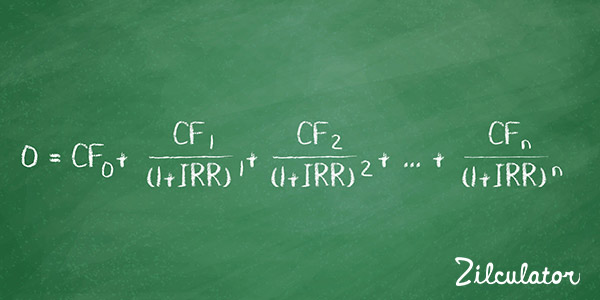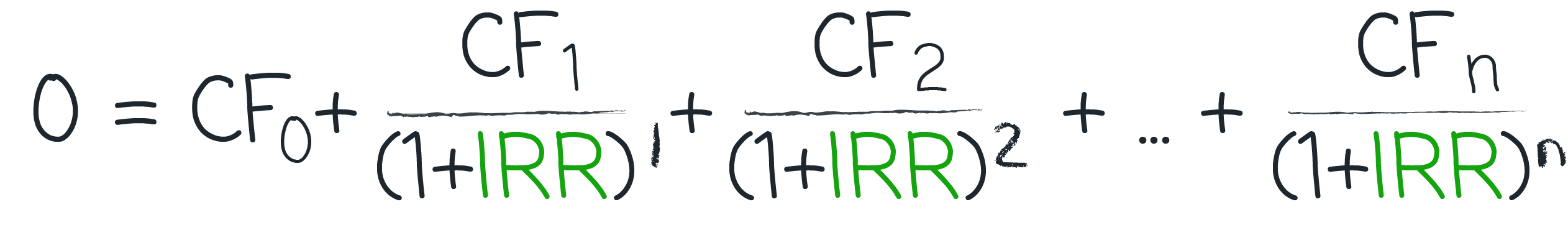# How to Calculate IRR

## Formula & DefinitionInternal rate of return (IRR) is probably the rate-of-return measurement most widely used by real estate professionals. It allows you to take into account both the timing and the magnitude of cash flows produced by your income-property investment.

It's not a silver bullet; no approach to investment decision-making is. And by no means should you use it to the exclusion of other measurements discussed in Zilculator's Real Estate Analysis Metrics section. Those others run a range from the simplistic to sophisticated, but virtually all of them can help fill in the whole picture. A property's operating expense ratios, for example, may not give you enough information by themselves to guide you to a purchase decision, but they still may tell you something you absolutely need to know.

If you read article about Discounted Cash Flow or Net Present Value, then you know that you can apply a particular discount rate - your required rate of return - to a series of future cash flows to determine what that income stream is worth today. If you rearrange that process, you can re-characterize the present value (PV) as your known present cost (i.e., the amount of cash you have to invest in order to make this purchase) and treat the discount rate (the rate of return) as your unknown.

In discounted cash flow you estimated the future cash flows and the proper discount rate and then used that information to calculate the PV of your cash investment.

Now, if you forecast the future cash flows and the PV (i.e., the amount) of your actual cash investment, you can calculate the discount rate, which you call the internal rate of return.

Note: In Zilculator software, we use Cash Flow After Taxes for the calculation of IRR.

Zilculator helps real estate professionals calculate IRR easily. Never use a spreadsheet again! Analyze your own property or create investment reports for your clients.

• Sales and Rental comps

## How to Calculate Internal Rate of Return

1. If you try to figure an IRR manually, you'll spend a great deal of time. The process is an iterative, successive-approximation technique (math-speak for trial and error) that starts with a guess and narrows its way to a conclusion. Instead, use the Microsoft Excel spreadsheet example that you can download below.
2. Note that if your project has negative cash flows in the future, then it may not be possible to solve for an IRR. The solution to this problem is a technique called modified internal rate of return (MIRR).
3. Mathematical formula for IRR is below: